## 让我们来创建后期效果-高动态光照渲染!123456789101112131415161718192021222324252627282930313233343536373839404142 kernel Lum < namespace : "cn.dreamfairy";     vendor : "dreamfairy";     version : 1; > {     input image4 baseTex;     input image4 bloomTex;     output pixel4 dsts;         //外部参数    parameter float fAvgLum     < minValue:float(0.1);         maxValue:float(0.5);         defaultValue:float(0.5);     >;     parameter float fDimmer     < minValue:float(0);         maxValue:float(1);         defaultValue:float(1);     >;     void     evaluatePixel()     {         float4 texCol = sampleNearest(baseTex,outCoord());         //计算平均亮度         float vLum = 0.27 * texCol.r + 0.67 * texCol.g + 0.06 * texCol.b;         //亮度缩放         float vLumScaled = fDimmer * vLum / fAvgLum;                 texCol = float4(texCol.r * vLumScaled, texCol.g * vLumScaled,         texCol.b * vLumScaled, texCol.a * vLumScaled);         texCol = texCol / (float4(1.0) + texCol);                 float4 texBloom = sampleNearest(bloomTex, outCoord());                 dsts = texBloom + texCol;     } }

## 让我们来创建后期效果-模糊!

var index : uint = step <= 1 ? 1 : (step - 1) * 3; var num : uint = 8 * index; 之后我们需要用拼接的方式来构造AGAL

 12345678910111213141516171819202122232425262728293031323334353637383940414243444546474849 private function getBlurAgal(step : uint, force : uint, coord : String, pTempIndex : int, pSaveIndex : int, pConstantOffset : int, pTextureIndex : int, constant : Vector., pInitIndex : int) : String         {             var data : Array = new Array();             var isFirstSave : Boolean = true;             var loopNum : uint = 0;             var stepX : Number = force / stage.stageWidth;             var stepY : Number = force / stage.stageHeight;             var index : uint = step <= 1 ? 1 : (step - 1) * 3;             var num : uint = 8 * index;             var tempIndex : int = pTempIndex;             var saveIndex : int = pSaveIndex;             var textureIndex : int = pTextureIndex;             var initIndex : int = pInitIndex;             var constantOffset : int = pConstantOffset;             constant = constant || new Vector.();             constant.length = 0;                         data.push("mov ft" + tempIndex + ", fc" + initIndex);                         //横向遍历             for(var i : int = -step; i <= step; i++)             {                 //纵向遍历                 for(var j : int = -step; j <= step; j ++)                 {                     //跳过中心区                     if(i == 0 && j == 0) continue;                     constant.push(i * stepX, j * stepY);                     data.push("add ft" + tempIndex + ".xy, " + coord + ".xy, fc" + constantOffset + (loopNum % 2 == 0 ? ".xy" : ".zw"));                     data.push("tex ft" + tempIndex + ", ft" + tempIndex + ", fs" + textureIndex + "<2d, repeat, linear, mipnone>");                     data.push(                         isFirstSave ? "mov ft" + saveIndex + ", ft" + tempIndex :                         "add ft" + saveIndex + ", ft" + saveIndex + ", ft" + tempIndex);                     isFirstSave = false;                     if(++loopNum % 2 == 0) constantOffset++;                 }             }                         data.push("div ft" + saveIndex + ".rgb, ft" + saveIndex + ".rgb, fc" + constantOffset + ".w");             data.push("mov oc, ft" + saveIndex);                         if(constant.length / 4 > 28){                 return getBlurAgal(1,1,coord,pTempIndex,pSaveIndex,pConstantOffset,pTextureIndex,constant,pInitIndex);             }                         constant.push(0,0,0,num);             return data.join("n");         }

var stepX : Number = force / stage.stageWidth;
var stepY : Number = force / stage.stageHeight;

data.push(“mov ft” + tempIndex + “, fc” + initIndex);

(loopNum % 2 == 0 ? “.xy” : “.zw”)

if(constant.length / 4 > 28)

data.push(
isFirstSave ? “mov ft” + saveIndex + “, ft” + tempIndex :
“add ft” + saveIndex + “, ft” + saveIndex + “, ft” + tempIndex);
isFirstSave = false;

var m_blurAgal : String = getBlurAgal(3, 1, “v0″, 0, 1, 1, 0, m_blurConstant, 0); fragmentProgram.assemble(Context3DProgramType.FRAGMENT, m_blurAgal);

Github地址: click me

## 在stage3D中实现后期处理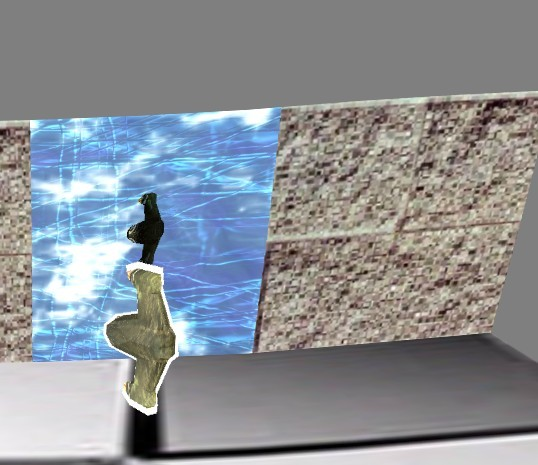1234567891011121314151617181920212223242526272829 private function renderPostProcessing() : void         {             // Render the scene to the scene texture             m_context.setRenderToTexture(m_sceneTexture, true);             renderCube();             m_context.setRenderToBackBuffer();             m_context.setProgram(m_normalShader);             m_context.setTextureAt(0, m_sceneTexture);             m_context.clear(0.5,0.5,0.5);             m_modelMatrix.identity();             m_modelMatrix.appendTranslation(0,0,1.21);             m_finalMatrix.identity();             m_finalMatrix.append(m_modelMatrix);             m_finalMatrix.append(m_viewMatrix);             m_finalMatrix.append(m_projMatrix);             m_context.setVertexBufferAt(0, m_sceneVertexBuffer, 0, Context3DVertexBufferFormat.FLOAT_3);             m_context.setVertexBufferAt(1,m_sceneVertexBuffer, 3, Context3DVertexBufferFormat.FLOAT_2);             m_context.setProgramConstantsFromMatrix(Context3DProgramType.VERTEX, 0, m_finalMatrix, true);             m_context.drawTriangles(m_sceneIndexBuffer,0,2);             m_context.setTextureAt(0,null);             m_context.setVertexBufferAt(0,null);             m_context.setVertexBufferAt(1,null);         }

m_context.setRenderToTexture(m_sceneTexture, true);

renderCube();

m_context.setRenderToBackBuffer();

Demo is Here : demo

## 3D世界物体拾取 续

 12345678910111213141516171819202122232425262728293031 /**          * 包围球射线检测          * param o 射线原点          * param d 射线角度          * param c 圆心          * param r 半径          */         public static function RaySphereIntersect(o : Vector3D, d : Vector3D, c : Vector3D, r : Number) : Boolean         {             var intersect : Boolean;             var s : Number;             var l : Vector3D = c.subtract(o); //圆心到射线原点的距离             s = d.dotProduct(l); //以射线起点开始,以射线的方向步进和包围球的长度的距离                         var ll : Number = l.length * l.length; //以射线的起点为圆心,以和包围球的距离为半径假设一个球形             var rr : Number = r * r;                         if(s < 0 && ll > rr) return false; //s < 0 表示目标在射线之后， ll > rr 表示射线不在圆内                         var mm : Number = ll - s*s; //圆心垂直于射线方向的长度             if(mm > rr) return false; //和射线构成的三角形不在圆内                         var q : Number = Math.sqrt(rr - mm); //圆心垂直于射线方向的点到射线碰撞的圆表面点的距离             var t : Number; //射线起点和圆相交点的距离             if(ll > rr)  //当射线在圆外                 t = s - q;             else                 t = s + q;                         return true;            }
 12345678910111213141516171819202122232425262728293031323334353637383940 stage.addEventListener(MouseEvent.CLICK, onClick);         private function onClick(e:MouseEvent) : void         {             //将鼠标点击位置偏移视口中央             var sceenX : Number = stage.mouseX;             var sceenY : Number = stage.mouseY;                         var viewX : Number = (sceenX * 2  / stage.stageWidth) - 1;             var viewY : Number = (-sceenY * 2 / stage.stageHeight) + 1;                         var ray : Ray = new Ray();             //将射线转换到相机所在空间             var viewMat : Matrix3D = m_camera.getViewMatrix().clone();             ray.origin = viewMat.transformVector(new Vector3D(0,0,0));                         //将相机反转,相机的平移和旋转总是和世界坐标系相反的             viewMat.invert();             ray.direction =  viewMat.deltaTransformVector(new Vector3D(viewX,viewY,1));             ray.direction.normalize();                         //取出相机的缩放值             var scale : Vector3D = m_proj.decompose();                         //将射线转到目标所在空间             var cubeMesh : CubeMesh;             for each(cubeMesh in m_cubeList)             {                 //相交检测                 var cubePos : Vector3D = cubeMesh.transform.position;                 cubePos.x *= scale.x;                 cubePos.y *= scale.y;                 cubePos.z *= scale.z;                 if(Ray.RaySphereIntersect(ray.origin, ray.direction, cubePos, 2))                     trace("碰撞", m_cubeList.indexOf(cubeMesh));             }         }

btw: 该来的总会来的,工作开始转向用cocos2d-x来制作手游了. stage3D这块还会继续研究的,只是成为副业了. o(︶︿︶)o 唉 真坑爹.

## 在stage3D中实现模型描边效果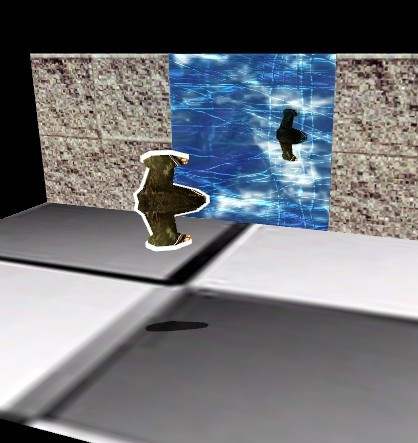Demo 地址：
Demo is here

## 在stage3D中行走在高低起伏的地形之上

 1 public function getHeight(x : Number, z : Number) : Number

 12345678910 x = (m_width >> 1) + x; z = (m_depth >> 1) - z; //地形上的x,y. 就是以地形的中心点为前提再偏移. m_width >> 1 和 m_depth >> 1 就是将xz平面的地方 / 2 后,取到中心点. x /= m_cellSpacing; z /= m_cellSpacing; var col : int = Math.floor(x); var row : int = Math.floor(z); //我们的地形的每个顶点的间距是 m_cellSpacing ,本demo中的值是10. /= 的方法就是求 col 和 row 的索引值,相信做过2D游戏A*寻路,或者地图区块平铺的同学知道.

 1234 var A : Number = getHeightMapEntry(row, col); var B : Number = getHeightMapEntry(row, col + 1); var C : Number = getHeightMapEntry(row + 1, col); var D : Number = getHeightMapEntry(row + 1, col + 1);

return m_heightMap[row * m_numVertsPerRow + col];

 1234567891011121314151617181920 var dx : Number = x - col; var dz : Number = z - row; //我们将当前位置偏移到这个平面的左上角             var height : Number = 0; if(dz < 1 - dx) //当 dz < 1-dx 时,表示我们在左上半部分的三角形上 {     var uy : Number = B - A;     var vy : Number = C - A;                     height = A + Utils.lerp(0,uy,1 - dx) + Utils.lerp(0,vy,1 - dz);         //计算这个三角形邻边和对边的线性插值.  Utils.lerp就是计算线性插值的函数. }else{ //当 dz > 1-dx 时,表示我们在右下半部分的三角形上     var uy : Number = C - D;     var vy : Number = B - D;                     height = D + Utils.lerp(0,uy,1 - dx) + Utils.lerp(0,vy,1 - dz); }             return height;

 1234 public static function lerp(a : Number, b : Number, t : Number) : Number {     return a - (a * t) + (b * t); }

## 在stage3D中创建一个海浪的效果

by the way~ 其实描边什么的我也都实现了,下周开始转向模型了.

## 在stage3D中拾取物体

Sx = Px(Width / 2) + X + Width / 2;

Sy = -Py(Height / 2) + Y + Height / 2;

[00 01 02 03]
[10 11 12 13]
[20 21 22 23]
[30 31 32 33]

 1234567891011121314151617 package {     import flash.geom.Vector3D;     public class Ray     {         /**起点**/         public var origin : Vector3D;                 /**方向**/         public var direction : Vector3D;                 public function Ray()         {         }     } }

 1234567891011121314151617181920 /**          * 计算射线          */         private function calcPicingRay(x : int, y : int) : Ray         {             var px : Number = 0;             var py : Number = 0;                         var vp : Rectangle = new Rectangle(0,0,stage.stageWidth,stage.stageHeight);                         var rawData : Vector. = m_proj.rawData;             px = (((2 * x) / vp.width) - 1.0) / rawData;             py = (((-2 * y) / vp.height) + 1.0) / rawData;                         var ray : Ray = new Ray();             ray.origin = new Vector3D(0,0,0);             ray.direction = new Vector3D(px,py,1);                         return ray;         }

 123456789101112131415 /**          * 转换射线          */         private function transformRay(ray : Ray, t : Matrix3D) : void         {             //转换射线的原点, w = 1             ray.origin.w = 1;             ray.origin = Utils.subjectMat(ray.origin,t,ray.origin);                         //转换射线的方向, w = 0;             ray.direction.w = 0;             ray.direction = Utils.subjectMat(ray.direction,t,ray.direction);                         ray.direction.normalize();         }

Utils.subjectMat 是我自己写的向量乘以矩阵的实现函数, 我不知道stage3D中是否有该API,如果有,请告知我是哪个. 这里我也提供下这个函数

 1234567891011121314 //向量左乘矩阵         public static function subjectMat(vec : Vector3D, mat : Matrix3D, out : Vector3D) : Vector3D         {             var data : Vector. = mat.rawData;                         if(null == out) out = new Vector3D();                         out.x = (vec.x * data + vec.y * data + vec.z * data + vec.w * data);             out.y = (vec.x * data + vec.y * data + vec.z * data + vec.w * data);             out.z = (vec.x * data + vec.y * data + vec.z * data + vec.w * data);             out.w = (vec.x * data + vec.y * data + vec.z * data + vec.w * data);                         return out;         }

||P-C|| – R = 0;

|| P0 + tU – C || – R = 0;

At^2 + Bt + C = 0; 这个公式很熟悉吧~ 貌似初中就学过了.
t0 = (-B + Sqrt(B * B – 4AC) / 2;
t1 = (-B – Sqrt(B * B – 4Ac) / 2;

 123456789101112131415161718192021222324 /**          * 碰撞检测          */         private function raySphereIntTest(ray : Ray, cube : CubeMesh) : Boolean         {             var v : Vector3D = ray.origin.subtract(cube.position);             var b : Number = 2 * ray.direction.dotProduct(v);             var c : Number = v.dotProduct(v) - (cube.radius * cube.radius);                         var discriminant : Number = (b * b) - (4 * c);                         if(discriminant < 0)                 return false;                         discriminant = Math.sqrt(discriminant);                         var s0 : Number = (-b + discriminant) / 2;             var s1 : Number = (-b - discriminant) / 2;                         if(s0 >= 0 || s1 >= 0)                 return true;                         return false;         }

 1234567891011121314 private function onClick(e:MouseEvent) : void         {             var ray : Ray = calcPicingRay(e.stageX,e.stageY);             var viewInverse : Matrix3D = m_viewMatrix.clone();             viewInverse.invert();             transformRay(ray,viewInverse);                         if(raySphereIntTest(ray,m_cubeList)){                 tip.text = "碰撞";             }               else{                 tip.text = "W,S,A,D 控制飞船移动n方向键控制相机移动";             }           }

Demo is Here

## 在stage3D中动态创建贴图并绘制地形阴影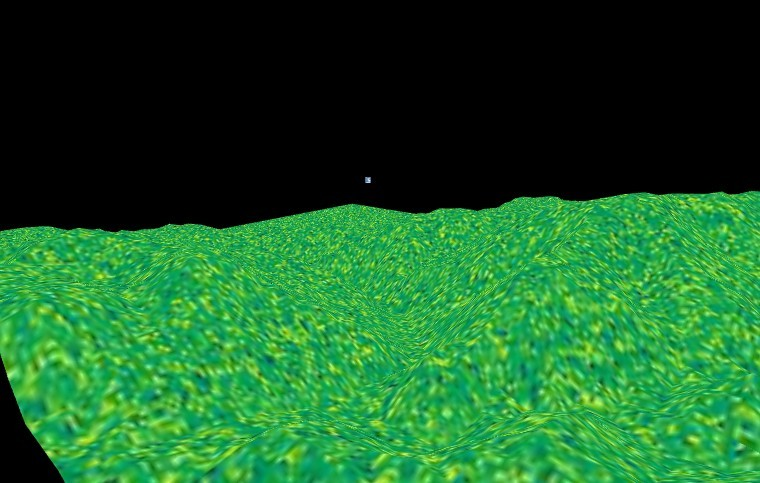123456789101112131415161718192021222324252627282930313233343536373839 public function genTexture(light : Vector3D) : void         {             var texWidth : int = m_numVertsPerRow;             var texHeight : int = m_numVertsPerCol;                         var emptyBmd : BitmapData = new BitmapData(texWidth, texHeight,false);             emptyBmd.lock();                         for(var i : int = 0; i < texHeight; i ++)             {                 for(var j : int = 0; j < texWidth; j++)                 {                     var c : uint;                     var height : Number = getHeightMapEntry(i,j) / m_heightScale;                                         if(height < 42.5) c = Utils.BEACH_SAND;                     else if(height < 85) c = Utils.LIGHT_YELLOW_GREEN;                     else if(height < 127.5) c = Utils.PUREGREEN;                     else if(height < 170) c = Utils.DARK_YELLOW_GREEN;                     else if(height < 212.5) c = Utils.DARKBROWN;                     else c = Utils.WHITE;                                         emptyBmd.setPixel32(j,i,c);                 }             }                         emptyBmd.unlock();             setTexture(emptyBmd);         }         public function getHeightMapEntry(row : int, col : int) : int         {             return m_heightMap[row * m_numVertsPerRow + col];         }         public function setTexture(data : BitmapData) : void         {             m_texture = Utils.getTextureByBmd(data, m_context3D);         }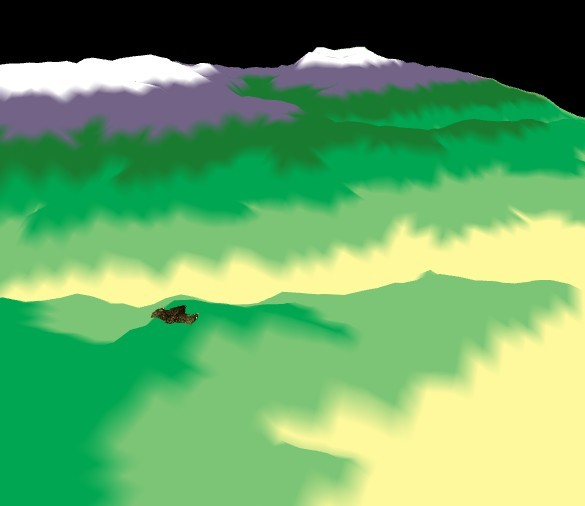## 在stage3D中使用高度图来绘制地形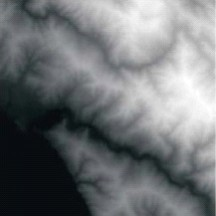12 [Embed(source="../../source/coastMountain64.raw",mimeType = "application/octet-stream")]         private var terrainData : Class;

 1234567891011 private function parseHeightMaps(data : ByteArray) : Boolean         {             if(null == data || 0 == data.bytesAvailable) return false;             var len : uint = data.bytesAvailable;             for(var i : int = 0; i < len; i++){                 m_heightMap.push(data.readUnsignedByte());             }                         return true;         }

 12 var uCoordIncrementSize : Number = 1.0 / 每列顶点数;             var vCoordIncrementSize : Number = 1.0 / 每行顶点数;

startZ = 0, endZ = 63;
startX = 0, endX = 63;

 123456789101112 for(z = startZ; z >= endZ; z -= m_cellSpacing)             {                 j = 0;                 for(x = startX; x <= endX; x += m_cellSpacing)                 {                     //计算当前顶点缓冲的索引，避免死循环                     var index : int = i * m_numVertsPerRow + j;                     m_rawVertex.push(x,m_heightMap[index],z,j * uCoordIncrementSize,i * vCoordIncrementSize);                     j++;                 }                 i++;             }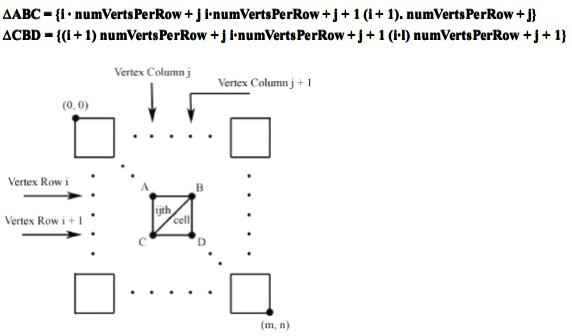123456789101112131415161718192021 private function computeIndices() : void         {             var baseIndex : int = 0;                         for(var i : int = 0; i < m_numCellsperCol; i++)             {                 for(var j : int = 0; j < m_numCellsPerRow; j++)                 {                     m_rawIndex[baseIndex]       = i * m_numVertsPerRow + j;                     m_rawIndex[baseIndex + 1]   = i * m_numVertsPerRow + j + 1;                     m_rawIndex[baseIndex + 2]   = (i + 1) * m_numVertsPerRow + j;                                         m_rawIndex[baseIndex + 3]   = (i + 1) * m_numVertsPerRow + j;                     m_rawIndex[baseIndex + 4]   = i * m_numVertsPerRow + j + 1;                     m_rawIndex[baseIndex + 5]   = (i + 1) * m_numVertsPerRow + j + 1;                                         baseIndex += 6;                 }             }             trace("索引解析完毕");         }

demo is here